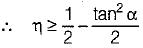Courses

# Threaded Joints And Power Screws - 3

## 20 Questions MCQ Test Topicwise Question Bank for Mechanical Engineering | Threaded Joints And Power Screws - 3

Description
This mock test of Threaded Joints And Power Screws - 3 for Mechanical Engineering helps you for every Mechanical Engineering entrance exam. This contains 20 Multiple Choice Questions for Mechanical Engineering Threaded Joints And Power Screws - 3 (mcq) to study with solutions a complete question bank. The solved questions answers in this Threaded Joints And Power Screws - 3 quiz give you a good mix of easy questions and tough questions. Mechanical Engineering students definitely take this Threaded Joints And Power Screws - 3 exercise for a better result in the exam. You can find other Threaded Joints And Power Screws - 3 extra questions, long questions & short questions for Mechanical Engineering on EduRev as well by searching above.
QUESTION: 1

Solution:
QUESTION: 2

### When a nut is tightened by placing a washer below it, the bolt will be subjected to

Solution:

Due to tightening bolt is always subjected to tensile stress.

QUESTION: 3

### Alien bolts are

Solution:
QUESTION: 4

The function of a washer is to

Solution:
QUESTION: 5

M 12 x 1.25 represents

Solution: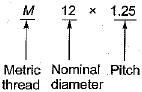If pitch is mentioned in the designation of th screw thread then, it is fine pitch otherwise coarse pitch.

QUESTION: 6

Eight bolts are to be selected for fixing the cover plate of a cylinder subjected to a maximum load of 980.175 kN. If the design stress for the bolt material is 315 N/mim2, what is the diameter of each bolt?

Solution:

Tensile force on each bolt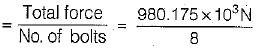for design of bolt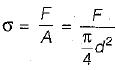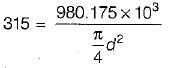d = 22.25 mm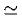30 mm (for safe design)

QUESTION: 7

Which one of the following is the value of helix angle for maximum efficiency of a square threaded screw? (φ = tan-1μ)

Solution:
QUESTION: 8

Which of the following screw thread is adopted for power transmission in either direction

Solution:

QUESTION: 9

To ensure self-locking in a screw jack it is essential that helix angle is

Solution:

For.self locking
φ ≤ α (Friction angle > Helix angle)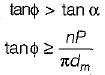(μ = tan φ)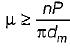QUESTION: 10

For overhauling condition

Solution:

If φ < α
i.e., friction angle is less then Helix angle, then torque require to lower the load will be negative i.e., no force is required to lower the load the ioad itself will begin to turn the screw and descend down, unless a restraining torque is applied this condition is known as overhauling.

QUESTION: 11

Solution:

1. It provides large axial motion per revolution of the screw, this increases the travelling speed of the sliding member.
2. The efficiency of multithreaded screw is more than single threaded screw due to increase in helix angle.
Note that the mechanical advantage obtained with multiple start threaded screw is lower than that of single threaded screw therefore the effort required to raise a particular load will be more in case of multiple start screw then single start.

QUESTION: 12

The maximum efficiency of seif locking screw is

Solution:

For self-locking, the maximum efficiency of screw should be 50%.
If efficiency is more than 50% the condition is known as overhauling.

QUESTION: 13

For a self-locking screw

Solution:

For self locking
φ ≤ a (Friction angle > Helix angle)
tan φ > tan α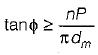(μ = tanφ)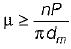QUESTION: 14

The main draw back of power screw is that

Solution:

Since in power screw, the seif locking property is desirable and to achieve self locking property, the power screw are designed for low efficiency.

QUESTION: 15

Solution:

2. Square threads are difficult to manufacture. They are usually turned on lathe with single point cutting tool.
3. The strength of a screw depends upon the thread thickness at the core diameter since square thread have less thickness at core than trapezoidal this reduces the load carrying capacity.

QUESTION: 16

If P is the pitch of a square thread, then the depth of thread d is given by

Solution: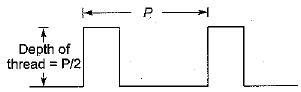QUESTION: 17

Screws used for power transmission should have

Solution:

Screw used for power transmission should have
1. High efficiency
2. High strength
4. Smooth and noise less

QUESTION: 18

Acme threads are practically used in

Solution:
QUESTION: 19

In case of power screws, what is the combination of materials used for the screw and the nut?

Solution:

Steel-screw, Phosphor Bronze-nut, Cast iron also used for nut in case of low speed.

QUESTION: 20

Consider the follow ing statem ents regarding power screws.
1. The efficiency of self locking screw cannot be more than 50%.
2. If friction angle is less than the helix angle of the screw, then the efficiency will be more than 50%.
3. The efficiency of ACME (Trapezoidal) thread is less than that of square thread.
Q. Which of these statements are correct?

Solution:

η less than 50% so statement 1 correct α > φ statements 2 incorrect